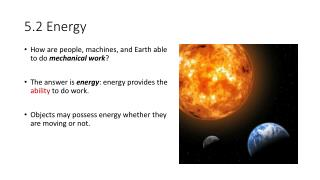Download Presentation5.2 Energy

# 5.2 Energy

Download Presentation## 5.2 Energy

- - - - - - - - - - - - - - - - - - - - - - - - - - - E N D - - - - - - - - - - - - - - - - - - - - - - - - - - -
##### Presentation Transcript

1. 5.2 Energy • How are people, machines, and Earth able to do mechanical work? • The answer is energy: energy provides the ability to do work. • Objects may possess energy whether they are moving or not.

2. 5.2 Kinetic Energy A moving object has the ability to do work because it can apply a force to another object and displace it. Kinetic Energy (EK) is the energy possessed by a moving object. A fast moving hammer has the ability to apply a force on a nail and push it into a piece of wood. The faster the hammer moves, the greater its kinetic energy, and the greater the displacement of the nail.

3. 5.2 Quantifying Kinetic Energy Consider a motorcycle moving in a straight line on a roadway with a total mass, m. The work equation is used to describe the relationship between the total work done on the motorcycle, the net force acting on it, its displacement, and the value of θ.

4. 5.2 Quantifying Kinetic Energy Using the 5 uniform acceleration equations from the kinematics unit:

5. 5.2 Quantifying Kinetic Energy Wnet is the total work done on the motorcycle to cause it to reach its final speed, v. It also equals the amount of kinetic energy the motorcycle has at speed v. Kinetic Energy is a scalar quantity; there is no direction associated with it. SI units ⤍ ⤍ Joule (J); 1 J = 1 N⋅m

6. 5.2 Work-Energy Principle This relationship between kinetic energy and total work is the work-energy principle. SP # 1 p.231; SP # 1 p.232 The total work done on an object (Wnet ) is a change in the object’s kinetic energy.

7. 5.2 Gravitational Potential Energy “The higher you go, the harder you fall.” An object positioned above the ground has the ability to fall and gain kinetic energy that could do mechanical work. It is able to fall because of the force of gravity acting on it. The ability of an object to do work because of the forces in its environment is called potential energy. It is considered a stored type of energy. Gravitation Potential Energy (Eg) is the energy possessed by an object based on its position relative to a reference level, which is often the ground. In order to determine the gravitational potential energy of an object, a designated reference level must be established where the object’s Eg= 0.

8. 5.2 Quantifying Gravitational Potential Energy The gravitational potential energy of an object of mass min kg, lifted to a height hin m, above a reference level, is given by the following equation: Gravitational Potential Energy is a scalar quantity; it has no direction. SI units ⤍Joule (J); 1 J = 1 N⋅m The work done by the construction worker on the hammer, in order to lift it, transfers energy to the hammer, increasing its gravitational potential energy. SP # 1 p.234

9. 5.2 Total Mechanical Energy Objects may posses kinetic energy (EK)only, gravitational potential energy (Eg)only, or a combination of both relative to some reference level. For example: • A hockey puck sliding on flat ice has kinetic energy only. • An apple hanging from a tree branch has gravitational potential energy only. • A parachutist falling from the sky has both kinetic and gravitational potential energy Total Mechanical Energy (ET)is the sum of an object’s kinetic and gravitational potential energy at a particular moment. ET = Eg+ EK

10. 5.2 Homework • Practice # 3 p.231 • Practice # 1,2 p.232 • Practice # 1 p.234 • Questions # 1-3, 5 p.235